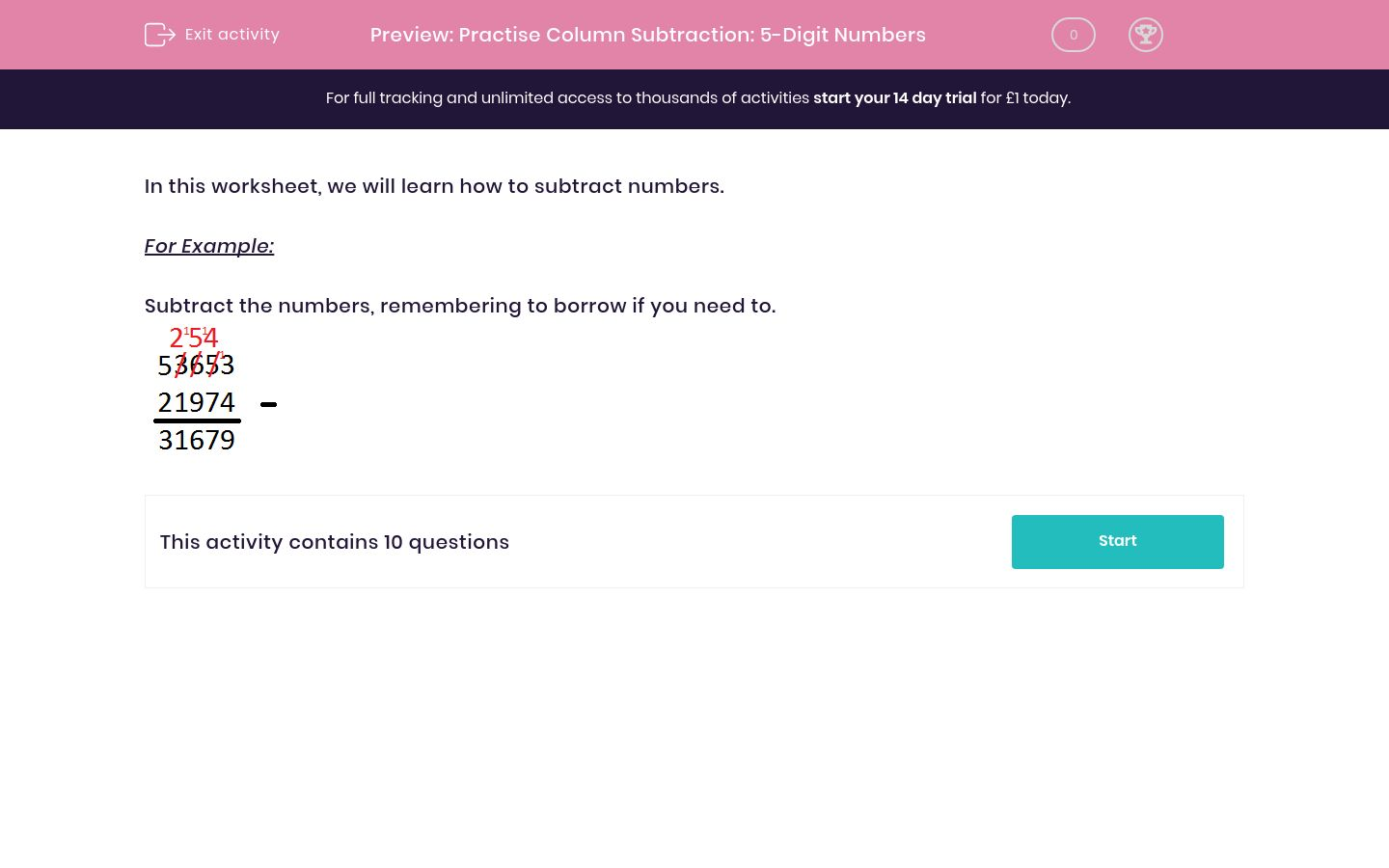# Practise Column Subtraction: 5-Digit Numbers

In this worksheet, students practise subtracting one five-digit number from another using columns.Key stage:  KS 2

Curriculum topic:   Maths and Numerical Reasoning

Curriculum subtopic:   Mixed Problems

Difficulty level:### QUESTION 1 of 10

In this worksheet, we will learn how to subtract numbers.

For Example:

Subtract the numbers, remembering to borrow if you need to.Subtract the numbers.

82382 – 23620 = ___________

Subtract the numbers.

37647 – 34597 = ___________

Subtract the numbers.

98358 – 68214 = ___________

Subtract the numbers.

81382 – 62986 = ___________

Subtract the numbers.

74609 – 14403 = ___________

Subtract the numbers.

92308 – 37452 = ___________

Subtract the numbers.

74941 – 31742 = ___________

Subtract the numbers.

64547 – 28538 = ___________

Subtract the numbers.

92952 – 75207 = ___________

Subtract the numbers.

31492 – 21194 = ___________

• Question 1

Subtract the numbers.

82382 – 23620 = ___________

58762
• Question 2

Subtract the numbers.

37647 – 34597 = ___________

3050
• Question 3

Subtract the numbers.

98358 – 68214 = ___________

30144
• Question 4

Subtract the numbers.

81382 – 62986 = ___________

18396
• Question 5

Subtract the numbers.

74609 – 14403 = ___________

60206
• Question 6

Subtract the numbers.

92308 – 37452 = ___________

54856
• Question 7

Subtract the numbers.

74941 – 31742 = ___________

43199
• Question 8

Subtract the numbers.

64547 – 28538 = ___________

36009
• Question 9

Subtract the numbers.

92952 – 75207 = ___________

17745
• Question 10

Subtract the numbers.

31492 – 21194 = ___________